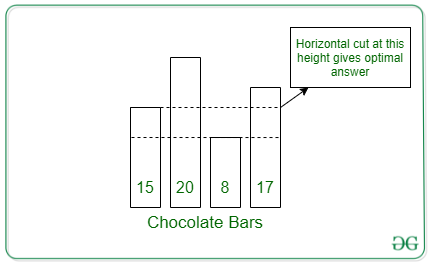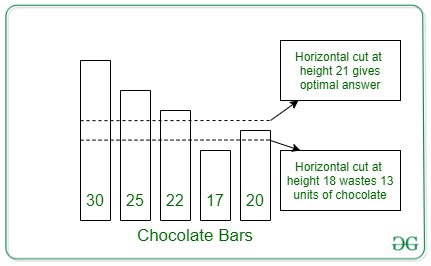Related Articles

# Find maximum height to cut all chocolates horizontally such that at least K amount remains

• Difficulty Level : Hard
• Last Updated : 17 Sep, 2021

Given an array arr[] consisting of heights of N chocolate bars, the task is to find the maximum height at which the horizontal cut is made to all the chocolates such that the sum remaining amount of chocolate is at least K.

Examples:

Input: K = 7, arr[] = [15, 20, 8, 17]
Output: 15
Explanation:If a cut is made at height 8 the total chocolate removed is 24 and chocolate wasted is 24 – 7 = 17 units. If a cut is made at height 15 then chocolate removed is 7 and no chocolate is wasted. Therefore 15 is the answer.

Input: K = 12 arr[] = [30, 25, 22, 17, 20]
Output: 21
Explanation:After a cut at height 18, the chocolate removed is 25 and chocolate wastage is (25 – 12) = 13 units. But if the cut is made at height 21 is made then 14 units of chocolate is removed and the wastage is (14 – 12) = 2 which is the least, hence 21 is the answer

Approach: The given problem can be solved based on Binary Search. The idea is to perform the Binary Search over then range [0, max element of the array] and find that value in the range that, say M such that the sum of remaining chocolate after making the horizontal cut at M give minimum difference with K. Follow the steps below to solve the given problem:

• Initialize two variables, say low and high as 0 and the maximum array element respectively.
• Iterate until low <= high and perform the following steps:
• Find the value of mid as (low + high)/2.
• Find the sum of remaining chocolates after making the horizontal cut at height mid as M.
• If the value of M is less than K, then update the value of high as (mid – 1). Otherwise, update the value of low as (mid + 1).
• After performing the above steps, print the value as high as the resultant maximum height that must be cut.

Below is the implementation of the above approach:

## C++

 `// C++ program for the above approach``#include``using` `namespace` `std;` `// Function to find the sum of remaining``// chocolate after making the horizontal``// cut at height mid``int` `cal(vector<``int``> arr, ``int` `mid)``{`` ` `      ``// Stores the sum of chocolates``    ``int` `chocolate = 0;``  ` `    ``// Traverse the array arr[]``    ``for``(``auto` `i : arr){``      ` `          ``// If the height is at least mid``        ``if``(i >= mid)``          ``chocolate += i - mid;``    ``}``        ` `            ` `    ``// Return the possible sum``    ``return` `chocolate;``}``    ` `// Function to find the maximum horizontal``// cut made to all the chocolates such that``// the sum of the remaining element is``// at least K``int` `maximumCut(vector<``int``> arr, ``int` `K){``  ` `      ``// Ranges of Binary Search``    ``int` `low = 0;``    ``int` `high = *max_element(arr.begin(),arr.end());``    ` `    ``// Perform the Binary Search``    ``while``(low <= high){``        ``int` `mid = (low + high) / 2;` `        ``// Find the sum of removed after``        ``// making cut at height mid``        ``int` `chocolate = cal(arr,mid);` `        ``// If the chocolate removed is``        ``// same as the chocolate needed``        ``// then return the height``        ``if` `(chocolate == K)``            ``return` `mid;` `        ``// If the chocolate removed is``        ``// less than chocolate needed``        ``// then shift to the left range``        ``else` `if``(chocolate < K)``            ``high = mid - 1;` `        ``// Otherwise, shift to the right``        ``// range``        ``else``{``            ``low = mid + 1;``            ``if``(mid > high)``                ``high = mid;``        ``}``    ``}    ``                ` `    ``// Return the possible cut``    ``return` `high;``}` `// Driver Code``int` `main(){``   ``int` `N = 4;``   ``int` `K = 7;``   ``vector<``int``> arr{15, 20, 8, 17};``   ``cout<<(maximumCut(arr, K));``}` `// This code is contributed by ipg2016107.`

## Java

 `// Java program for the above approach``import` `java.util.*;``import` `java.util.Arrays;``class` `GFG {` `    ``// Function to find the sum of remaining``    ``// chocolate after making the horizontal``    ``// cut at height mid``    ``static` `int` `cal(``int` `arr[], ``int` `mid)``    ``{` `        ``// Stores the sum of chocolates``        ``int` `chocolate = ``0``;` `        ``// Traverse the array arr[]``        ``for` `(``int` `i = ``0``; i < arr.length; i++) {` `            ``// If the height is at least mid``            ``if` `(arr[i] >= mid)``                ``chocolate += arr[i] - mid;``        ``}` `        ``// Return the possible sum``        ``return` `chocolate;``    ``}` `    ``// Function to find the maximum horizontal``    ``// cut made to all the chocolates such that``    ``// the sum of the remaining element is``    ``// at least K``    ``static` `int` `maximumCut(``int` `arr[], ``int` `K)``    ``{` `        ``// Ranges of Binary Search``        ``int` `low = ``0``;``        ``int` `high = Arrays.stream(arr).max().getAsInt();` `        ``// Perform the Binary Search``        ``while` `(low <= high) {``            ``int` `mid = (low + high) / ``2``;` `            ``// Find the sum of removed after``            ``// making cut at height mid``            ``int` `chocolate = cal(arr, mid);` `            ``// If the chocolate removed is``            ``// same as the chocolate needed``            ``// then return the height``            ``if` `(chocolate == K)``                ``return` `mid;` `            ``// If the chocolate removed is``            ``// less than chocolate needed``            ``// then shift to the left range``            ``else` `if` `(chocolate < K)``                ``high = mid - ``1``;` `            ``// Otherwise, shift to the right``            ``// range``            ``else` `{``                ``low = mid + ``1``;``                ``if` `(mid > high)``                    ``high = mid;``            ``}``        ``}` `        ``// Return the possible cut``        ``return` `high;``    ``}` `    ``// Driver Code``    ``public` `static` `void` `main(String[] args)``    ``{``        ``int` `K = ``7``;``        ``int` `arr[] = { ``15``, ``20``, ``8``, ``17` `};``        ``System.out.println(maximumCut(arr, K));``    ``}``}` `// This code is contributed by ukasp.`

## Python3

 `# Python program for the above approach` `# Function to find the sum of remaining``# chocolate after making the horizontal``# cut at height mid``def` `cal(arr, mid):``  ` `      ``# Stores the sum of chocolates``    ``chocolate ``=` `0``    ` `    ``# Traverse the array arr[]``    ``for` `i ``in` `arr:``      ` `          ``# If the height is at least mid``        ``if` `i >``=` `mid:``            ``chocolate ``+``=` `i ``-` `mid``            ` `    ``# Return the possible sum``    ``return` `chocolate` `# Function to find the maximum horizontal``# cut made to all the chocolates such that``# the sum of the remaining element is``# at least K``def` `maximumCut(arr, K):``  ` `      ``# Ranges of Binary Search``    ``low ``=` `0``    ``high ``=` `max``(arr)``    ` `    ``# Perform the Binary Search``    ``while` `low <``=` `high:``        ``mid ``=` `(low ``+` `high) ``/``/` `2` `        ``# Find the sum of removed after``        ``# making cut at height mid``        ``chocolate ``=` `cal(arr, mid)` `        ``# If the chocolate removed is``        ``# same as the chocolate needed``        ``# then return the height``        ``if` `chocolate ``=``=` `K:``            ``return` `mid` `        ``# If the chocolate removed is``        ``# less than chocolate needed``        ``# then shift to the left range``        ``elif` `chocolate < K:``            ``high ``=` `mid ``-` `1` `        ``# Otherwise, shift to the right``        ``# range``        ``else``:``            ``low ``=` `mid ``+` `1``            ``if` `mid > high:``                ``high ``=` `mid``                ` `    ``# Return the possible cut``    ``return` `high` `# Driver Code``N, K ``=` `4``, ``7``arr ``=` `[``15``, ``20``, ``8``, ``17``]` `print``(maximumCut(arr, K))`

## C#

 `// C# program for the above approach``using` `System;``using` `System.Collections.Generic;``using` `System.Linq;` `class` `GFG{` `// Function to find the sum of remaining``// chocolate after making the horizontal``// cut at height mid``static` `int` `cal(List<``int``> arr, ``int` `mid)``{`` ` `      ``// Stores the sum of chocolates``    ``int` `chocolate = 0;``  ` `    ``// Traverse the array arr[]``    ``foreach` `(``int` `i ``in` `arr){``      ` `          ``// If the height is at least mid``        ``if``(i >= mid)``          ``chocolate += i - mid;``    ``}``        ` `            ` `    ``// Return the possible sum``    ``return` `chocolate;``}``    ` `// Function to find the maximum horizontal``// cut made to all the chocolates such that``// the sum of the remaining element is``// at least K``static` `int` `maximumCut(List<``int``> arr, ``int` `K){``  ` `      ``// Ranges of Binary Search``    ``int` `low = 0;``    ``int` `high = arr.Max();``    ` `    ``// Perform the Binary Search``    ``while``(low <= high){``        ``int` `mid = (low + high) / 2;` `        ``// Find the sum of removed after``        ``// making cut at height mid``        ``int` `chocolate = cal(arr,mid);` `        ``// If the chocolate removed is``        ``// same as the chocolate needed``        ``// then return the height``        ``if` `(chocolate == K)``            ``return` `mid;` `        ``// If the chocolate removed is``        ``// less than chocolate needed``        ``// then shift to the left range``        ``else` `if``(chocolate < K)``            ``high = mid - 1;` `        ``// Otherwise, shift to the right``        ``// range``        ``else``{``            ``low = mid + 1;``            ``if``(mid > high)``                ``high = mid;``        ``}``    ``}    ``                ` `    ``// Return the possible cut``    ``return` `high;``}` `// Driver Code``public` `static` `void` `Main(){``   ``int` `K = 7;``   ``List<``int``> arr = ``new` `List<``int``>(){15, 20, 8, 17};``   ``Console.Write(maximumCut(arr, K));``}` `}` `// This code is contributed by SURENDRA_GANGWAR.`

## Javascript

 ``

Output:
`15`

Time Complexity: O(N*log N)
Auxiliary Space: O(1)

Attention reader! Don’t stop learning now. Get hold of all the important DSA concepts with the DSA Self Paced Course at a student-friendly price and become industry ready.  To complete your preparation from learning a language to DS Algo and many more,  please refer Complete Interview Preparation Course.

In case you wish to attend live classes with experts, please refer DSA Live Classes for Working Professionals and Competitive Programming Live for Students.

My Personal Notes arrow_drop_up